# qPCR on ChIPed chromatin

The quality of the immunoprecipitated material is usually evaluated by qPCR before being converted into sequencing library. This is the aim of this section.

## Principle of the analysis:

The Bio-Rad CFX Real-Time PCR detection system will be used with pairs of primers used in the practicals have been validated beforehand.

All qPCR quantifications are done in triplicates.

### Calculation of Amplification efficiency = primer efficiency

RAW data (output of CFX analysis software)

• B01/B02/B03, B04/B05/B06 and B07/B08/B09 are standard dilution (1:5 dilution ratio) of a DNA samples (three triplicates)
• Cq is given by the qPCR machine
• Cq Mean is the mean Cq of the three triplicates
• The log (Qty) correspond to the log value of the standard

Using the RAW data, a standard curve can be plotted and the qPCR amplification efficiency calculated as follows: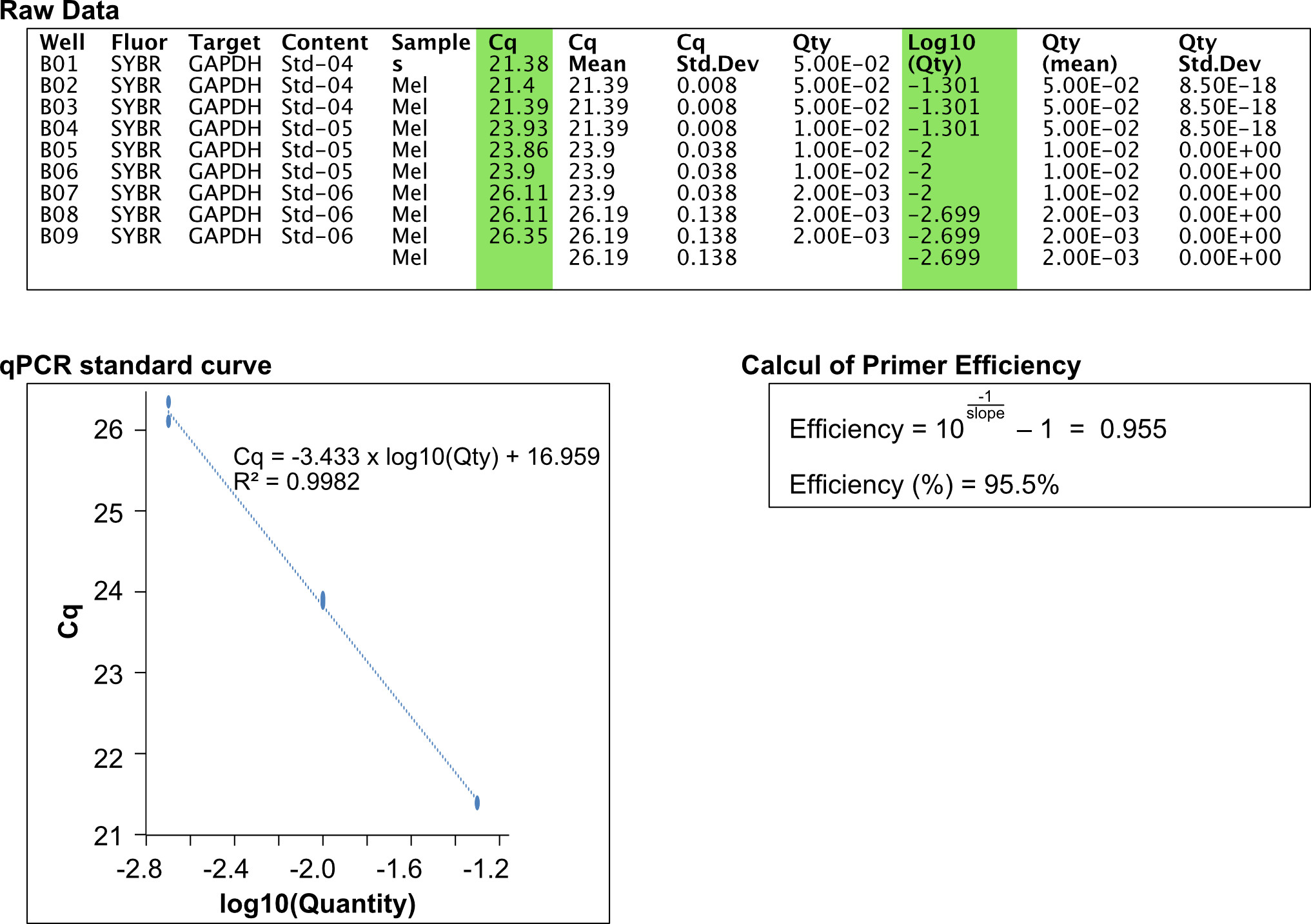### Calculation of “Percent of Input”

The “Percent of Input” is calculted using the Mean Cq value, primer efficiency and Input dilution. This is illustrated below for two primer pairs and 3 different IPs including one with a control GFP antibody.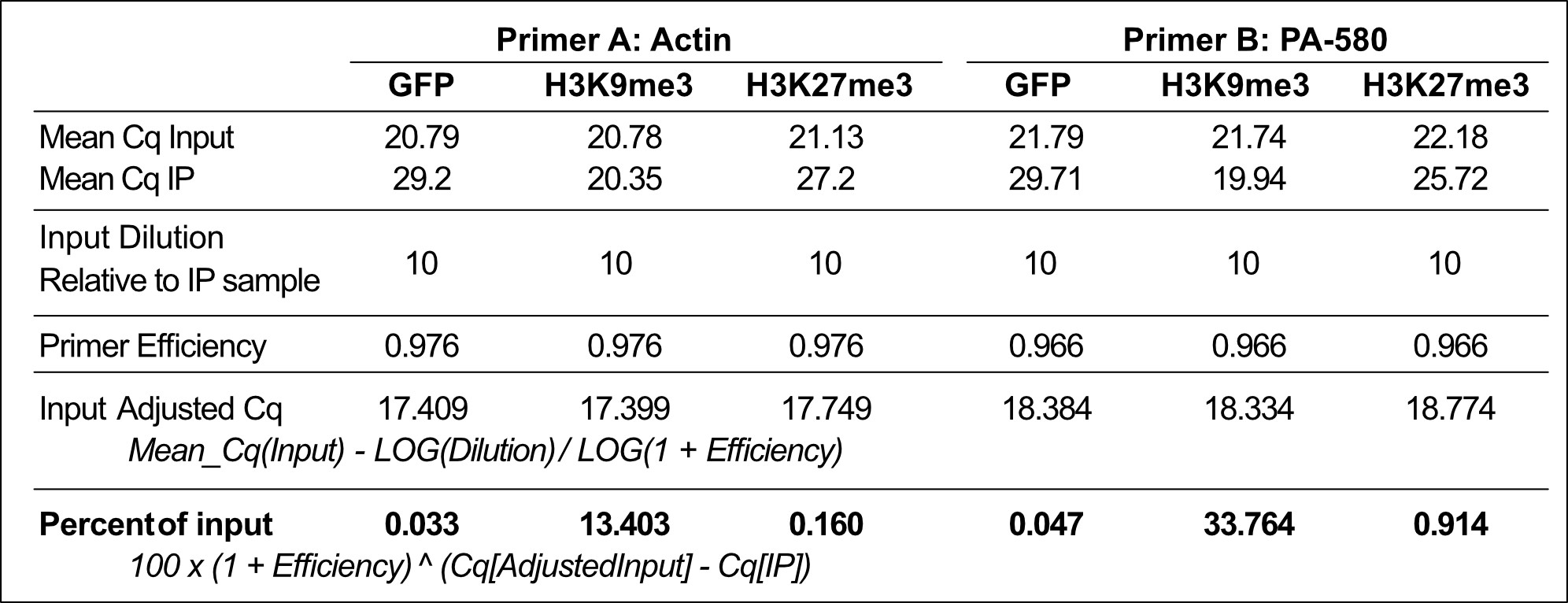## Implementation for the practical

### DNA dilution

——📝🔴—– Benoit: 5/07/2022: Last year, we used a 1:5 dilution, instead of a 1:20. Should we upadate (a) the sentence below and (b) the calculations in the different tables? ——📝—–

You can test different DNA dilutions to come across the right Cq (20-22) or do like me and test 1:20 which in my opinion corresponded to a good approximation of reality.

In theory, the Cq of the Samples should not differ from the Input more than a factor of 4, but I consider that we are OK if we stay in that range (avoid exceeding a Sample>30)

### qPCR mix

Prepare a master mix for n+3 reactions (if you have 20 qPCR reaction to do, the mix should be prepared for 23 reactions)

• qPCR mix:
• 5μl of 2X MasterMix
• 2μl H2O
• 1μl of primer mix 5μM
• 2μl of diluted DNA

### qPCR reactions to do

• Beforehand, we need to quantify primer efficiency for each primer pair:
• We need to prepare a “standard” by mixing different DNA as follows:
• 1 volume of your diluted Input (6µL)
• 0.5 volume of your diluted IP1 (3µL)
• 0.5 volume of your diluted IP2 (3µL)
• Make a serial dilution (1:5) this “standard” (2µL into 8µL H2O). 3 different dilutions are enough. careful: the acurracy of the serial dilution is essential.
• These 3 dilutions are referred to as “std-1”, “std-2” and “std-3” in the layout for the qPCR.
• These PCR are used to determine primer efficiency (see below).

——📝🔴—– Benoit: 5/07/2022: Last year, we used a 1:5 dilution, instead of a 1:20. Should we upadate (c) the sentences below ——📝—–

• qPCR reactions:
• The three standards. In triplicates
• A single Input diluted 1/20 (normally it’s the same for each antibody but you can test them all before selecting one). In triplicates
• All your IP Samples diluted 1/20 (or adequate dilution). In triplicates
• Include two Negative control wells (i.e. wells in which DNA replaced by H2O)
• [In same cases, we also need to PCR a plate reporter point (Known DNA concentration, always the same pair of antibodies between each plate) (optional: useful to compare samples on different runs). We won’t need it here.]
• Plot measured Cq as a function of the log10(quantity) of the 3 standards. This allows to determine qPCR efficiency (see above) and detect the presence of potential PCR inhibitors.

• In total, for each primer pair:
• For the calculation of primer efficiency: 3 x 3 = 9 wells
• negative controls (H2O): 2 wells
• For the calculation of “Percent of Input”: 3 wells Input + 3 wells IP1 + 3 wells of IP2 = 9 wells.
• An optional “reporter point” (3 wells)
Figure - Layout for the qPCR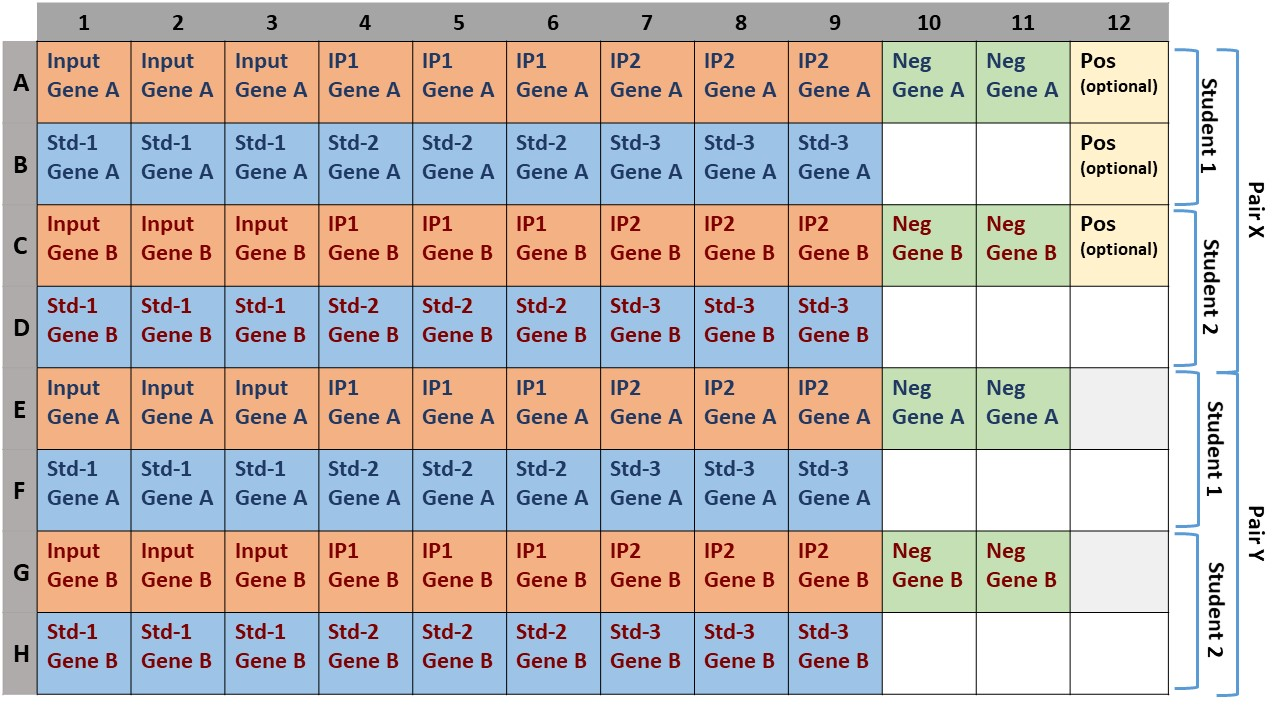## Analysis of the results:

The analysis and the different calculation can be done using the provided excel file: Excel file

Adjust the Cq of the input: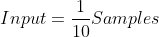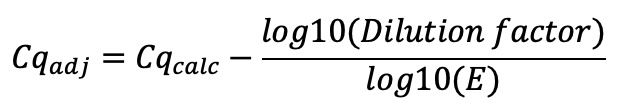Where E stands for the primer efficiency.

Adjustment of the Cq_Input (for the same dilution in qPCR):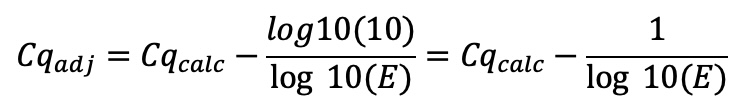For each Sample: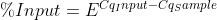In addition, you can also calculate the standard deviation for this enrichment with the following formula:whereall the formulas will be explained during the workshop